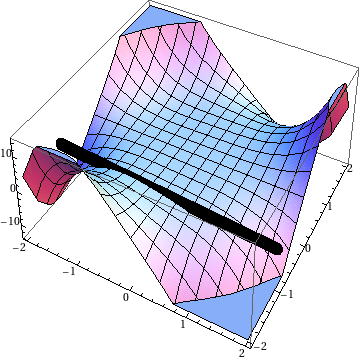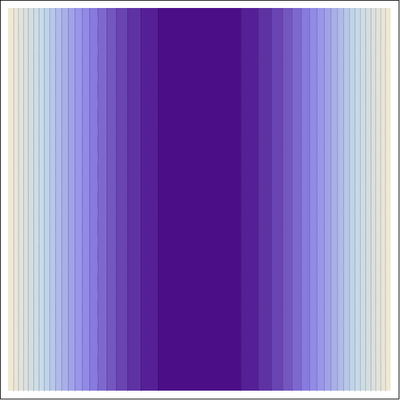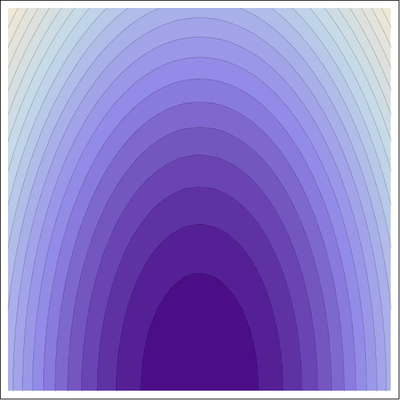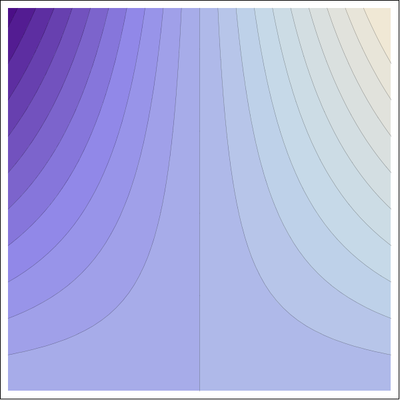Math 21a, Fall 2008
Exhibits page Math 21a 2008, Multivariable Calculus
The meaning of the mixed partial derivative fxyAssume we have a function f(x,y) of two variables like f(x,y) = x2 y. The partial derivative fx is the rate of change of the function f in the x direction. We also can see that xx means: it is positive if the surface is bent concave up in the x direction and negative if it is bent concave down in the x direction. What it the interpretation of the derivative fxy? One can interpret it as the rate of change of the slope in the x-direction as one moves into the y direction.